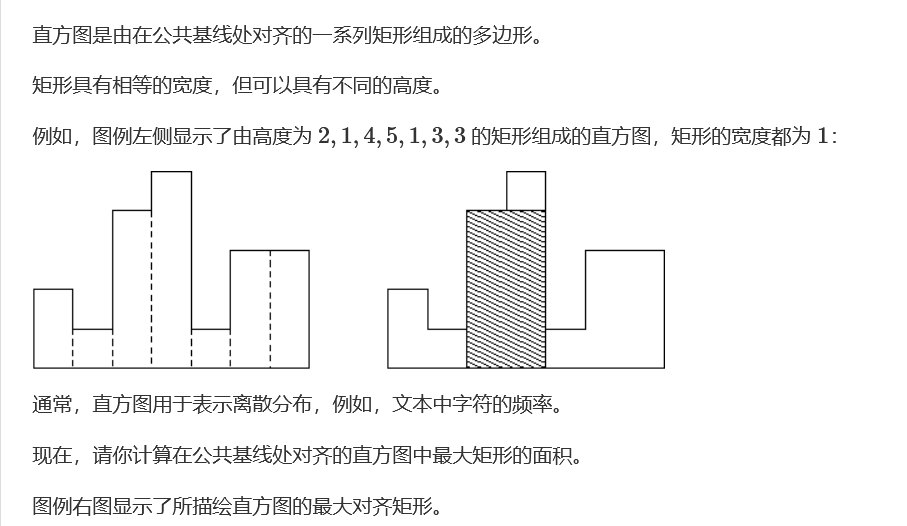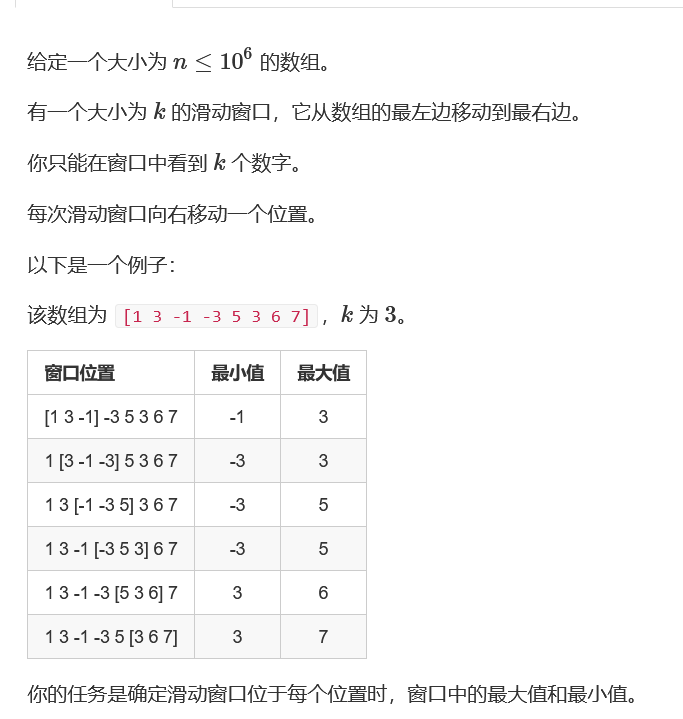8.18-8.20单调队列单调栈

2021/8/21 23:53:38 浏览：

单调栈

直方图中最大的矩形#include<iostream>
#include<algorithm>
#include<stack>
using namespace std;
typedef long long ll;
typedef pair<ll,int> pa;
const int N=1e5+10;
int n;
ll l[N],r[N],h[N];
void get(ll a[])
{
stack<pa> q;
h=-1;
q.push({-1,0});//防止栈空
for(int i=1;i<=n;i++)
{
while(q.top().first>=h[i])
q.pop();
a[i]=i-q.top().second;
q.push({h[i],i});
}
}
int main()
{
while(cin>>n&&n)
{
for(int i=1;i<=n;i++)
cin>>h[i];
get(l);
reverse(h+1,h+n+1);
get(r);
ll ans=0;
for(int i=1;i<=n;i++)
{
// cout<<r[n-i+1]+l[i]-1<<' '<<h[n-i+1]<<endl;
ans=max(ans,(r[n-i+1]+l[i]-1)*h[n-i+1]);
}
cout<<ans<<endl;
}
return 0;
}

小组队列

#include<iostream>
#include<algorithm>
#include<queue>
#include<map>
using namespace std;

map<int,int> book;
int main()
{
int t;
int num=0;
while(cin>>t&&t)
{
queue<int> q;
num++;
cout<<"Scenario #"<<num<<endl;
for(int i=1;i<=t;i++)
{
int n;
cin>>n;
while(n--)
{
int x;
cin>>x;
book[x]=i;
}
}
string op;
while(cin>>op)
{
if(op=="ENQUEUE")
{
int x;
cin>>x;
if(q[book[x]].empty())
q.push(book[x]);
q[book[x]].push(x);
}
else if(op=="DEQUEUE")
{
int xx=q.front();
cout<<q[xx].front()<<endl;
q[xx].pop();
if(q[xx].empty()) q.pop();
}
else if(op=="STOP")
{
cout<<endl;
break;
}
}
}
return 0;
}

双端队列

1．新建一个双端队列，并将当前数作为这个队列中的唯一的数；

2．将当前数放入已有的队列的头之前或者尾之后。

#include<iostream>
#include<algorithm>
using namespace std;
typedef long long ll;
typedef pair<ll,ll> pa;
const int N=2e5+10;
pa a[N];
int main()
{
int n;
cin>>n;
for(int i=1;i<=n;i++)
{
cin>>a[i].first;
a[i].second=i;
}
sort(a+1,a+1+n);
bool book=0;
ll pre=1e18;
ll ans=1;
for(int i=1;i<=n;i++)
{
int j=i+1;
while(a[j].first==a[i].first)
j++;
int mina=a[i].second,maxa=a[j-1].second;
// cout<<pre<<' '<<mina<<' '<<maxa<<endl;
if(!book)
{
if(pre>maxa)
pre=mina;
else
{
pre=maxa;
book=1;
}
}
else if(book)
{
if(mina>pre)
pre=maxa;
else
{
book=0;
ans++;
pre=mina;
}
}
i=j-1;
}
cout<<ans<<endl;
return 0;
}

单调队列

滑动窗口#include<iostream>
#include<algorithm>
using namespace std;
const int N=1e6+10;
int a[N],q[N],n,m;
int main()
{
cin>>n>>m;
int h=0,t=-1;
for(int i=0;i<n;i++)
{
cin>>a[i];
if(i-q[h]>=m) h++;
while(t>=h&&a[q[t]]>=a[i]) t--;
q[++t]=i;
if(i+1>=m) cout<<a[q[h]]<<' ';
}
cout<<endl;
h=0,t=-1;
for(int i=0;i<n;i++)
{
if(i-q[h]>=m) h++;
while(t>=h&&a[q[t]]<=a[i]) t--;
q[++t]=i;
if(i+1>=m) cout<<a[q[h]]<<' ';
}
return 0;
}

最大子序和

#include<iostream>
#include<algorithm>
using namespace std;
const int N=3e5+10;
int s[N],q[N];
int main()
{
int n,m;
cin>>n>>m;
for(int i=1;i<=n;i++)
{
cin>>s[i];
s[i]+=s[i-1];
}
int ans=-1e9;
int h=0,t=-1;
for(int i=0;i<=n;i++)
{
if(t>=h&&i-q[h]>m) h++;
if(i)ans=max(ans,s[i]-s[q[h]]);
while(t>=h&&s[q[t]]>=s[i]) t--;
q[++t]=i;
}
cout<<ans<<endl;
return 0;
}

堆

超市

#include<iostream>
#include<algorithm>
using namespace std;
typedef pair<int,int> pa;
const int N=1e4+10;
int f[N];
pa a[N];
int find(int k)
{
if(f[k]==k) return k;
return f[k]=find(f[k]);
}
int main()
{
int n;
while(cin>>n)
{
int d=0;
for(int i=1;i<=n;i++)
{
cin>>a[i].first>>a[i].second;
d=max(d,a[i].second);
}
for(int i=1;i<=d;i++)
f[i]=i;
sort(a+1,a+n+1);
int ans=0;
for(int i=n;i>0;i--)
{
int r=find(a[i].second);
if(r)
{
ans+=a[i].first;
f[r]=r-1;
}
}
cout<<ans<<endl;
}
return 0;
}